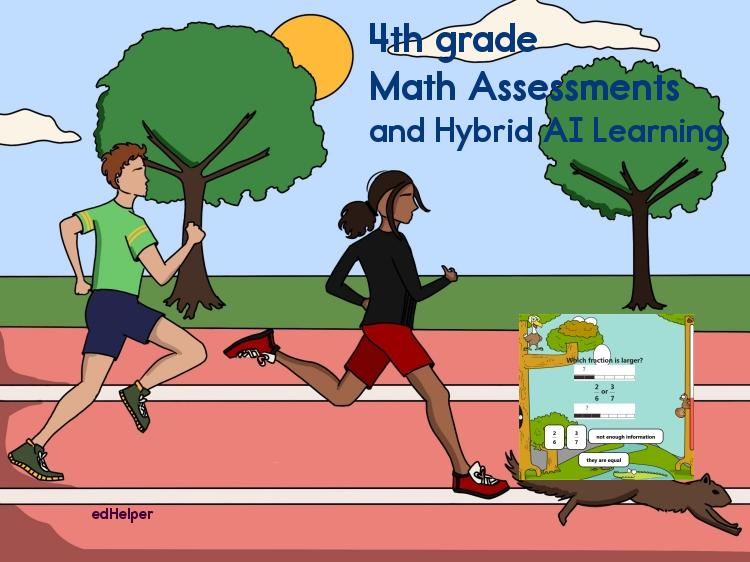# Math Assessments and Hybrid AI Learning (4th grade)Mixed Math Assessment Workbooks (with Online Math Practice Pages):
Sept-December 4th grade Math Assessments Workbook

January-May 4th grade Math Assessments Workbook

4th grade Math Assessments Workbook - an entire year of mixed math skills

Math Assessments:
Place Value and Large Numbers (easier)

Subtraction (easier)

Whole Numbers and Place Value

Clocks and Time (easier)

Multiplication

Fractions (intro)

Division

Estimation and Number Theory

Multiplying and Dividing by 1-Digit

Fractions (easier)

Fractions

Fractions and Decimals

Decimals